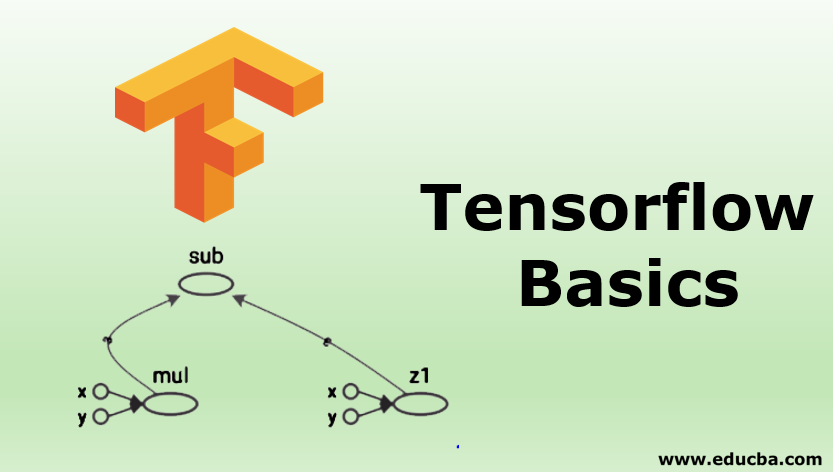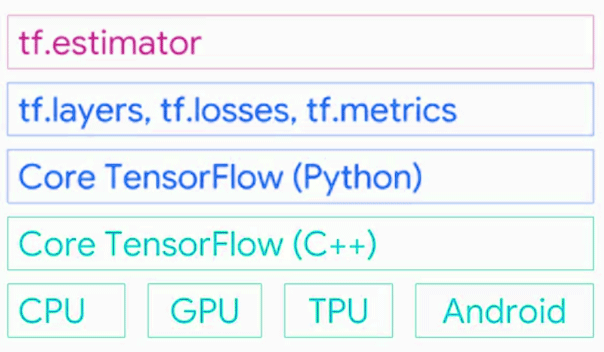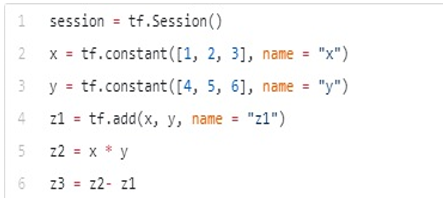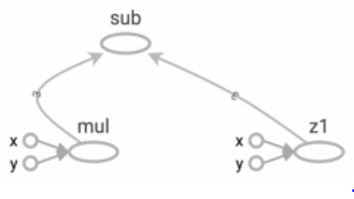# Tensorflow BasicsIntroduction to Tensorflow

Tensorflow is an open-source software and library. It was developed by Google Brain team which was built by deep learning artificial intelligence research team at google 2010. Google was using it for internal use after that it was released under Apache2.0 Open source – 2015. In this topic, we are going ot learn about Tensorflow Basics.

Tensorflow is google brain’s second-generation system. Version 1 was released on Feb 11, 2017. Tensorflow 1.0 now had Python API and API for java and GO language is also added to version 1.0. Tensorflow operations neural network performed on multidimensional data array, which is referred to as a tensor. It works with Tensors. It is a software library for deep learning and mainly works for numerical computation using data flow graphs.

What is Tensorflow Basics?

Tensors are objects that describe the linear relation between vectors, scalars, and other tensors. Tensors are nothing but multidimensional arrays.

Tensorflow provides support to write the code according to your requirements and access to different kinds of tools. We can write code C++ and can call C++ code from python. Or we can write python code and call it by C++.Seen above image the lowest layer it supports two languages first is Python language and second C++ language. You can write it in any language in your comfort zone. It has a collection of different math libraries that help to easily create math functions.

It also provides support for processing like CPU, GPU, TPU and also runs on android mobiles.

Tf.layers:- tf.layers is used for method abstract so you can customize the layers of neural networks.

Tf.estimator:- most commonly used API in tensorflow is the tf.estimator. It helps in create and train, test your model.

Installation of Tensorflow

First check your pip version, if not latest then run following command to upgrade pip

Run below code to install the simpler version of Tensorflow

pip install tensorflow / conda install tensorflow (Anaconda)

This will install Tensorflow with gpu supported configurations.

pip install Tensorflow-gpu

Example of tf.estimator to use classifier by 3 lines of code

Import tensorflow as tf
classifier = tf.estimator.LinearClassifier(feature_columns)
classifier.train(input_fn=train_input_function, steps=2000)
predictions = classifier.predict(input_fn=predict_input_function)

Basic Data types of Tensorflow

The basic data types in Tensorflow framework (Tensors)

Below shows each dimension of tensors.

Scalar – O Dimensional Array
Vector – 1 Dimensional Array
Matrix – 2 Dimensional Array
3D Tensor – 3 Dimensional Array
N – D Tensor – N-dimensional array

Constant Tensors
Variables

tf.Variable class, for creating a variable in tensorflow and calling the tf.get_variable function

Initializing Variables

To initialize the variables, By calling tf.global_variables_initializer we can initialize all the variables.

A simple example of variable and Math expression

Normal way

a = 3.0, b = 8*a +10

Tensorflow way

c = tf.Variable (tf.add (tf.multiply (X, a), b)

Graphs

Every line in our code written in tensorflow is converted into an underlying chart

Example:Nodes: It represents mathematical operations.
Edges: it represents the multidimensional array (Tensors) and shows how they communicate between them.

Tensorflow 2.0

In the second version of Tensorflow, they focused on making the API simpler and easy to use.
The API components integrate better with Keras, by default, the eager execution mode is activated.
Eager Mode:  Eager execution is run interface where operations are executed immediately as they are called from Python.
We can use eager mode instead of graph mode. We can compute what we need to compute and we can get results right away. This will make Tensorflow as easy as Pytorch
Focusing on remove duplication APIs.

Keras

Tensorflow provides a high-level API for building and training deep learning models. This was not included in tensorflow but in the latest release, Keras has been included in Tensorflow 2.0.
User-friendly: Keras provides a simple, consistence interface for common use cases.
Modular and composable: Keras models are made by connecting building blocks together.
Easy to extend: Create or update new layers, metrics, loss functions
Use tf.keras for using Keras models.

Tensorflow Lite

In 2017, Google announced software that is specifically built for mobile development, Tensorflow Lite.
Tensorflow Lite (TFLite) is a lightweight solution for on- Mobile device inference.
We can also use it for IOS and Android by creating C++ API, as well as we can also Java wrapper class for Android Developers.

List of Algorithm that Tensorflow supports
1. For Regression

Linear Regression (tf.estimator. Linear Regression)
Booster tree Regression (tf.estimator. Boosted Tree Regressor)

2. For Classification

Classification (tf.estimator. Linear Classifier)
Deep Learning Combined (tf.estimator. DNNLinearCombinedClassifier)
Boosted tree classifier (tf.estimator. Boosted Tree Classifier)

Features of Tensorflow

Tensorflow works Efficiently with different types of mathematical expressions involving Multidimensional arrays(Tensors)
It also provides support for deep learning neural networks and other machine learning concepts.
Tensorflow can run on multiple CPUs and GPUs.
It also provides its own processing power that is the Tensor Processing Unit.

Tensor Processing Unit (TPU)

Google announced its Tensor processing unit(TPU) an application-specific integrated circuit(Hardware Chip), built specifically for Machine Learning and tailored for Tensorflow.
In 2017, Google announced the second version of Tensorflow, as well as the availability of the TPUs in google cloud.
TPU is a programmable AI accelerator and built for using or running models. Google running TPUs inside their data centers for more than a year.

Edge TPU

Edge TPU is a chip that googles built for designed and run Tensorflow Lite machine learning (ML) models to run on small computing devices such as smartphones.
High scalability for computing huge datasets
It can also train and serve models in a live model. Rewriting of code I not required

Conclusion – Tensorflow Basics

Tensorflow is a very commonly used deep learning library. That is mostly used in creating neural networks, also used by startup companies, big companies.

As talked above Google is also using tensorflow for his internal purpose it still used in mostly all kinds of products like Gmail and google search engine.

Recommended Articles

This is a guide to Tensorflow Basics. Here we discuss the Installation of Tensorflow with the features and List of Algorithm That Tensorflow supports. You may also look at the following article to learn more –

The post Tensorflow Basics appeared first on EDUCBA.

## Like it? Share with your friends!Cry
0
CryCute
0
CuteDamn
0
DamnDislike
0
DislikeLike
0
LikeLol
0
LolLove
0
LoveWin
0
WinWTF
0
WTF

## Tensorflow Basics

Become a part of our community!Don't have an account?

Back to

Join BoomBox CommunityBack to